# P-space

$P$-space or $p$-space refers to various classes of topological space, discussed below.

PSPACE or $\mathcal{P}$-space refers to an algorithmic complexity class.

##-space in the sense of Gillman–Henriksen.

A-space as defined in [a2] is a completely-regular space in which every point is a-point, i.e., every fixed prime ideal in the ring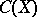of real-valued continuous functions is maximal (cf. also Maximal ideal; Prime ideal); this is equivalent to saying that every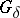-subset is open (cf. also Set of type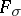()). The latter condition is used to define-spaces among general topological spaces. In [a5] these spaces were called-additive, because countable unions of closed sets are closed.

Non-Archimedean ordered fields are-spaces, in their order topology; thus,-spaces occur in non-standard analysis. Another source of-spaces is formed by the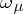-metrizable spaces of [a5]. Ifis a regular cardinal number (cf. also Cardinal number), then an-metrizable space is a setwith a mappingfrom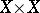to the ordinal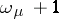that acts like a metric:if and only if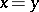;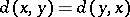and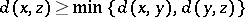;is called an-metric. A topology is formed, as for a metric space, using-balls: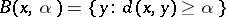, where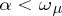. The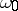-metrizable spaces are exactly the strongly zero-dimensional metric spaces [a8] (cf. also Zero-dimensional space). Ifis uncountable, then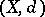is a-space (and conversely).

One also employs-spaces in the investigation of box products [a7]. If a productis endowed with the box topology, then the equivalence relation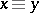defined by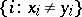being finite defines a quotient space of, denoted, that is a-space. The quotient mapping is open and the box product and its quotient share many properties.

##-space in the sense of Morita.

A-space as defined in [a3] is a topological spacewith the following covering property: Letbe a set and let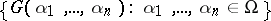be a family of open sets (indexed by the set of finite sequences of elements of). Then there is a familyof closed sets such that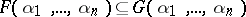and whenever a sequence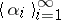satisfies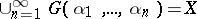, then also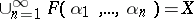. K. Morita introduced-spaces to characterize spaces whose products with all metrizable spaces are normal (cf. also Normal space): A space is a normal (paracompact)-space if and only if its product with every metrizable space is normal (paracompact, cf. also Paracompact space).

Morita [a4] conjectured that this characterization is symmetric in that a space is metrizable if and only if its product with every normal-space is normal. K. Chiba, T.C. Przymusiński and M.E. Rudin [a1] showed that the conjecture is true if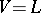, i.e. Gödel's axiom of constructibility, holds (cf. also Gödel constructive set). These authors also showed that another conjecture of Morita is true without any extra set-theoretic axioms: Ifis normal for every normal space, thenis discrete: cf. Morita conjectures.

There is a characterization of-spaces in terms of topological games [a6]; let two players, I and II, play the following game on a topological space: player I chooses open sets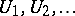and player II chooses closed sets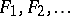, with the proviso that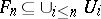. Player II wins the play if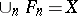. One can show that Player II has a winning strategy if and only ifis a-space.

## $p$-space in the sense of Arkhangel'skii.

A feathered space or plumed space, a completely-regular Hausdorff space having a feathering in some Hausdorff compactification, has been termed a $p$-space. For paracompact spaces these coincide with the $p$-spaces of Morita, [b1].

How to Cite This Entry:
P-space. Encyclopedia of Mathematics. URL: http://encyclopediaofmath.org/index.php?title=P-space&oldid=41942
This article was adapted from an original article by K.P. Hart (originator), which appeared in Encyclopedia of Mathematics - ISBN 1402006098. See original article